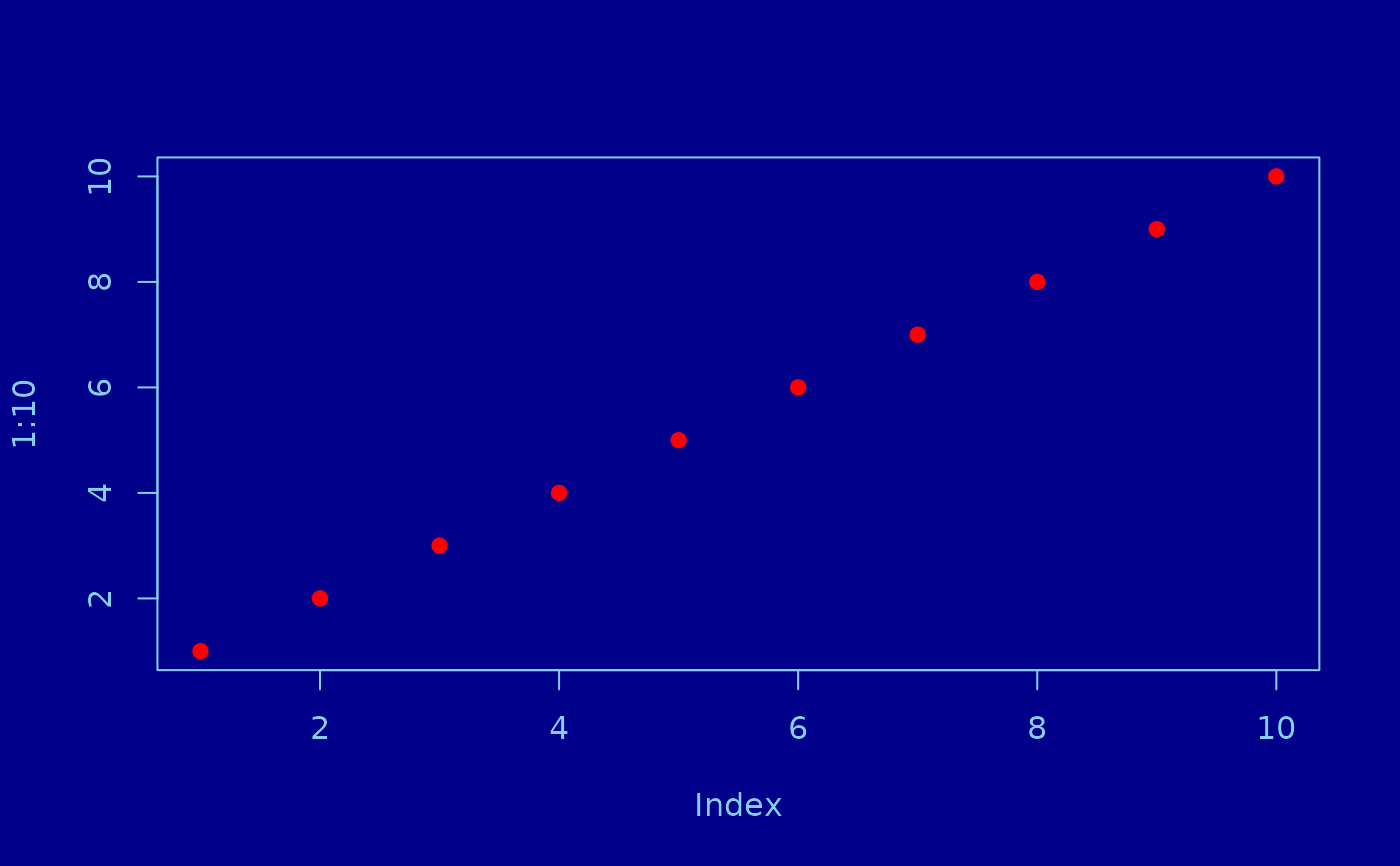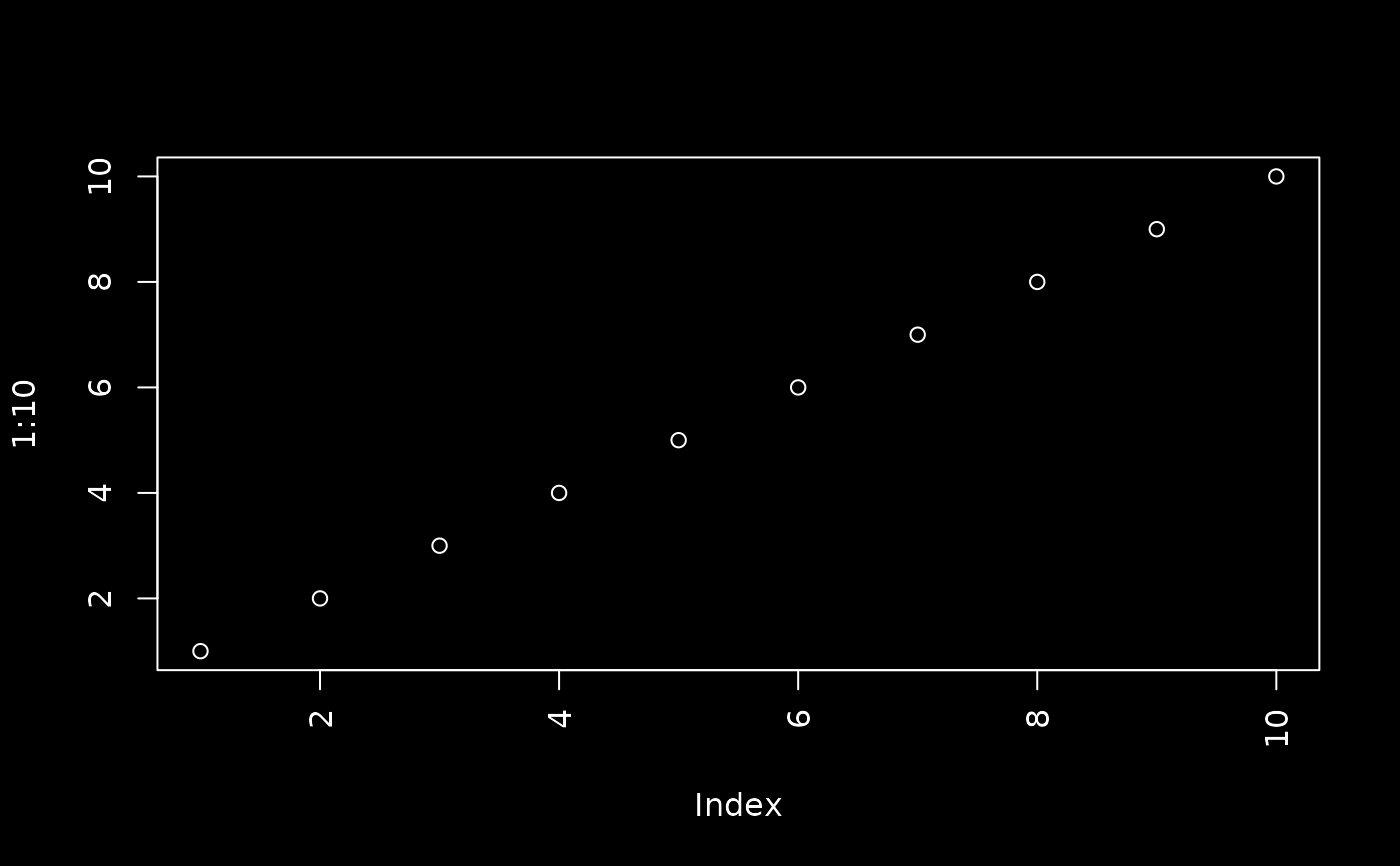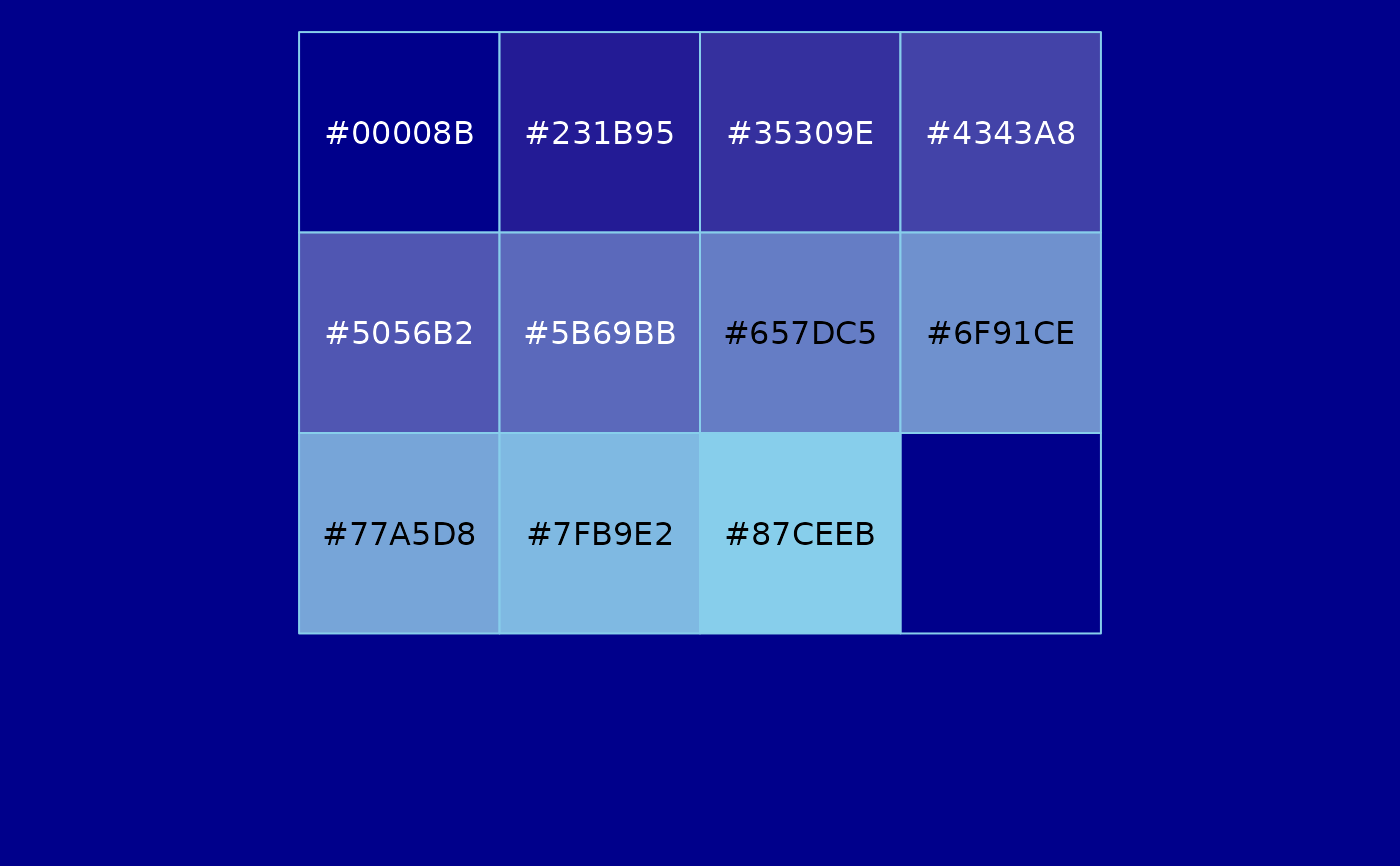These functions are helpful for getting and/or temporarily activating a thematic_theme().

thematic_with_theme(theme, expr)

thematic_local_theme(theme, .local_envir = parent.frame())

thematic_set_theme(theme)

thematic_get_theme(resolve = TRUE)

thematic_get_option(name = "", default = NULL, resolve = TRUE)

thematic_get_mixture(amounts = 0.5, default = NULL)

## Arguments

theme a thematic_theme() object (or a return value of thematic_on/thematic_get_theme()) or NULL (in which case thematic_off() is called). R code that produces a plot. The environment to use for scoping. whether or not 'auto' values should be resolved before returning a theme element name (e.g., fg, bg, etc.) a default value to return in the event no thematic theme is active. value(s) between 0 and 1 specifying how much to mix bg (0) and fg (1).

## Value

the result of expr.

## Functions

• thematic_with_theme: similar to thematic_on(), but for an single plot.

• thematic_local_theme: similar to thematic_with_theme(), but de-couples the theme from the plot expression.

• thematic_set_theme: set a given theme object as the current theme.

• thematic_get_theme: obtain the current theme.

• thematic_get_option: obtain a particular theme option (and provide a default if no theme is active).

• thematic_get_mixture: obtain a mixture of the current theme's bg and fg.

## Examples


# Use thematic_with_theme() for a one-time use of thematic
thematic_with_theme(
thematic_theme("darkblue", "skyblue", accent = "red"),
plot(1:10, col = thematic_get_option("accent"), pch = 19)
)# Use thematic_set_theme() if doing something more complicated
# like programming on top thematic (without causing side effects)
my_plot <- function(expr, las = 3, ...) {
old_theme <- thematic_on("black", "white")
on.exit(thematic_set_theme(old_theme), add = TRUE)
opts <- par(las = las)
on.exit(par(opts), add = TRUE)
# Imagine some more customization with ...
force(expr)
}
my_plot(plot(1:10))thematic_off()
thematic_get_option("bg", "white")
#>  "white"thematic_on(bg = "red")
thematic_get_option("bg", "white")
#> Warning: thematic was unable to resolve fg='auto'. Try providing an actual color (or NA) to the fg argument of thematic_on(). By the way, 'auto' is only officially supported in shiny::renderPlot(), some rmarkdown scenarios (specifically, html_document() with theme!=NULL), in RStudio, or if auto_config_set() is used.#>  "red"thematic_off()

thematic_with_theme(
thematic_theme("darkblue", "skyblue"),
scales::show_col(thematic_get_mixture(seq(0, 1, by = 0.1)))
)